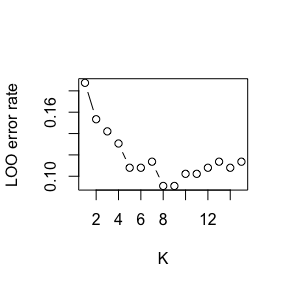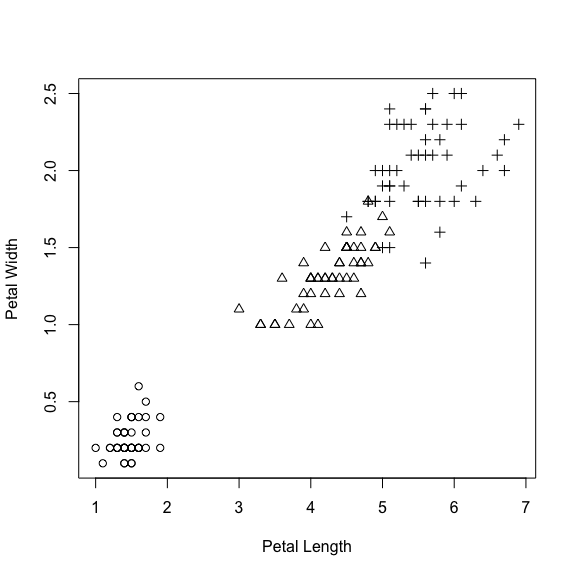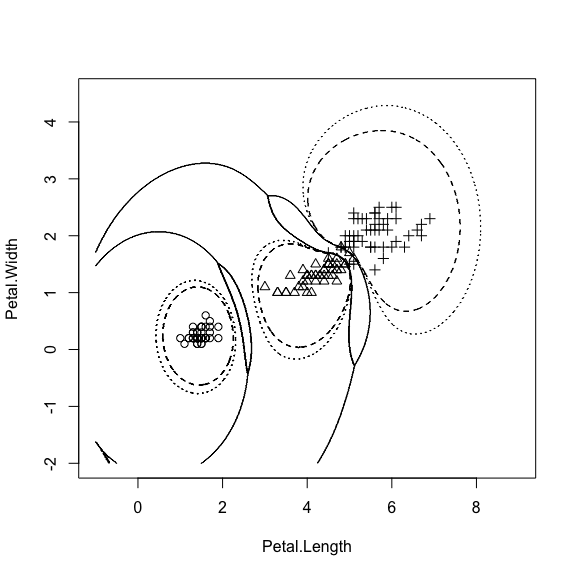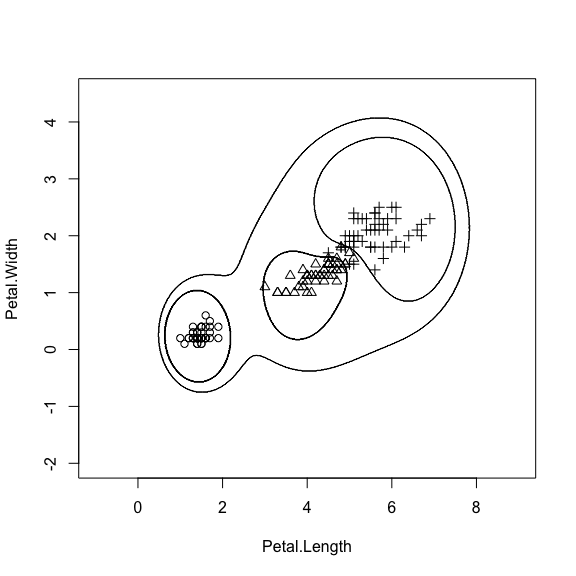# Introduction to the evclass package

#### 2017-03-19

The package evclass contains methods for evidential classification. An evidential classifier quantifies the uncertainty about the class of a pattern by a Dempster-Shafer mass function. In evidential distance-based classifiers, the mass functions are computed from distances between the test pattern and either training pattern, or prototypes. The user is invited to read the papers cited in this vignette to get familiar with the main concepts underlying evidential clustering. These papers can be downloaded from the author’s web site, at https://www.hds.utc.fr/~tdenoeux. Here, we provide a short guided tour of the main functions in the evclass package. The two classification methods implemented to date are:

• The evidential K-nearest neighbor classifier (Denœux 1995, Zouhal and Denœux (1998));
• The evidential neural network classifier (Denœux 2000).

You first need to install this package:

library(evclass)

The following sections contain a brief introduction on the way to use the main functions in the package evclass for evidential classification.

## Evidential K-nearest neighbor classifier

The principle of the evidential K-nearest neighbor (EK-NN) classifier is explained in (Denœux 1995), and the optimization of the parameters of this model is presented in (Zouhal and Denœux 1998). The reader is referred to these references. Here, we focus on the practical application of this method using the functions implemented in evclass.

Consider, for instance, the ionosphere data. This dataset consists in 351 instances grouped in two classes and described by 34 numeric attributes. The first 175 instances are training data, the rest are test data. Let us first load the data, and split them into a training set and a test set.

data(ionosphere)
xtr<-ionosphere$x[1:176,] ytr<-ionosphere$y[1:176]
xtst<-ionosphere$x[177:351,] ytst<-ionosphere$y[177:351]

The EK-NN classifier is implemented as three functions: EkNNinit for initialization, EkNNfit for training, and EkNNval for testing. Let us initialize the classifier and train it on the ionosphere data, with $$K=5$$ neighbors. (If the argument param is not passed to EkNNfit, the function EkNNinit is called inside EkNNfit; here, we make the call explicit for clarity).

param0<- EkNNinit(xtr,ytr)
options=list(maxiter=300,eta=0.1,gain_min=1e-5,disp=FALSE)
fit<-EkNNfit(xtr,ytr,param=param0,K=5,options=options)

The list fit contains the optimized parameters, the final value of the cost function, the leave-one-out (LOO) error rate, the LOO predicted class labels and the LOO predicted mass functions. Here the LOO error rate and confusion matrix are:

print(fit$err) ##  0.1079545 table(fit$ypred,ytr)
##    ytr
##       1   2
##   1 108  14
##   2   5  49

We can then evaluate the classifier on the test data:

val<-EkNNval(xtrain=xtr,ytrain=ytr,xtst=xtst,K=5,ytst=ytst,param=fit$param) print(val$err)
##  0.1142857
table(val$ypred,ytst) ## ytst ## 1 2 ## 1 107 15 ## 2 5 48 To determine the best value of $$K$$, we may compute the LOO error for different candidate value. Here, we will all values between 1 and 15 err<-rep(0,15) i<-0 for(K in 1:15){ fit<-EkNNfit(xtr,ytr,K,options=list(maxiter=100,eta=0.1,gain_min=1e-5,disp=FALSE)) err[K]<-fit$err
}
plot(1:15,err,type="b",xlab='K',ylab='LOO error rate')The minimum LOO error rate is obtained for $$K=8$$. The test error rate and confusion matrix for that value of $$K$$ are obtained as follows:

fit<-EkNNfit(xtr,ytr,K=8,options=list(maxiter=100,eta=0.1,gain_min=1e-5,disp=FALSE))
val<-EkNNval(xtrain=xtr,ytrain=ytr,xtst=xtst,K=8,ytst=ytst,param=fit$param) print(val$err)
##  0.09142857
table(val$ypred,ytst) ## ytst ## 1 2 ## 1 106 10 ## 2 6 53 ## Evidential neural network classifier In the evidential neural network classifier, the output mass functions are based on distances to protypes, which allows for faster classification. The prototypes and their class-membership degrees are leanrnt by minimizing a cost function. This function is defined as the sum of an error term and, optionally, a regularization term. As for the EK-NN classifier, the evidential neural network classifier is implemented as three functions: proDSinit for initialization, proDSfit for training and proDSval for evaluation. Let us demonstrate this method on the glass dataset. This data set contains 185 instances, which can be split into 89 training instances and 96 test instances. data(glass) xtr<-glass$x[1:89,]
ytr<-glass$y[1:89] xtst<-glass$x[90:185,]
ytst<-glass$y[90:185] We then initialize a network with 7 prototypes: param0<-proDSinit(xtr,ytr,nproto=7,nprotoPerClass=FALSE,crisp=FALSE) and train this network without regularization: options<-list(maxiter=500,eta=0.1,gain_min=1e-5,disp=20) fit<-proDSfit(x=xtr,y=ytr,param=param0,options=options) ##  1.0000000 0.3582862 10.0000000 ##  21.0000000 0.2933959 1.2426062 ##  41.0000000 0.2631432 0.1972797 ##  61.00000000 0.20079817 0.04424406 ##  81.00000000 0.19174016 0.01050067 ##  1.010000e+02 1.888532e-01 2.519525e-03 ##  1.210000e+02 1.885120e-01 6.370615e-04 ##  1.410000e+02 1.883220e-01 1.752994e-04 Finally, we evaluate the performance of the network on the test set: val<-proDSval(xtst,fit$param,ytst)
print(val$err) ##  0.3020833 table(ytst,val$ypred)
##
## ytst  1  2  4
##    1 31  9  0
##    2  5 28  4
##    3  5  3  0
##    4  0  3  8

If the training is done with regularization, the hyperparameter mu needs to be determined by cross-validation.

## Decision

In the belief function framework, there are several definitions of expectation. Each of these definitions results in a different decision rule that can be used for classification. The reader is referred to (Denœux 1997) for a detailed description of theses rules and there application to classification. Here, we will illustrate the use of the function decision for generating decisions.

We consider the Iris dataset from the package datasets. To plot the decisions regions, we will only use two input attributes: ‘Petal.Length’ and ‘Petal.Width’. This code plots of the data and trains the evidential neural network classifiers with six prototypes:

data("iris")
x<- iris[,3:4]
y<-as.numeric(iris[,5])
c<-max(y)
plot(x[,1],x[,2],pch=y,xlab="Petal Length",ylab="Petal Width")param0<-proDSinit(x,y,6)
fit<-proDSfit(x,y,param0)
##   1.0000000  0.3100244 10.0000000
##  11.0000000  0.1793266  3.5712815
##  21.00000000  0.07456371  1.31427661
##  31.0000000  0.0416460  0.4797702
##  41.00000000  0.03069473  0.19345069
##  51.00000000  0.03034766  0.07089198
##  61.00000000  0.02361211  0.02867826
##  71.00000000  0.02245256  0.01320300
##  81.000000000  0.021613541  0.005711898
##  91.000000000  0.021163977  0.002272939

Let us assume that we have the following loss matrix:

L=cbind(1-diag(c),rep(0.3,c))
print(L)
##      [,1] [,2] [,3] [,4]
## [1,]    0    1    1  0.3
## [2,]    1    0    1  0.3
## [3,]    1    1    0  0.3

This matrix has four columns, one for each possible act (decision). The first three decisions correspond to the assignment to each the three classes. The losses are 0 for a correct classification, and 1 for a misclassification. The fourth decision is rejection. For this act, the loss is 0.3, whatever the true class. The following code draws the decision regions for this loss matrix, and three decision rules: the minimization of the lower, upper and pignistic expectations (see (Denœux 1997) for details about these rules).

xx<-seq(-1,9,0.01)
yy<-seq(-2,4.5,0.01)
nx<-length(xx)
ny<-length(yy)
Dlower<-matrix(0,nrow=nx,ncol=ny)
Dupper<-Dlower
Dpig<-Dlower
for(i in 1:nx){
X<-matrix(c(rep(xx[i],ny),yy),ny,2)
val<-proDSval(X,fit$param) Dupper[i,]<-decision(val$m,L=L,rule='upper')
Dlower[i,]<-decision(val$m,L=L,rule='lower') Dpig[i,]<-decision(val$m,L=L,rule='pignistic')
}

contour(xx,yy,Dlower,xlab="Petal.Length",ylab="Petal.Width",drawlabels=FALSE)
for(k in 1:c) points(x[y==k,1],x[y==k,2],pch=k)
contour(xx,yy,Dpig,xlab="Petal.Length",ylab="Petal.Width",drawlabels=FALSE,add=TRUE,lty=3)As suggested in (Denœux 1997), we can also consider the case where there is an unknown class, not represented in the learning set. We can then construct a loss matrix with four rows (the last row corresponds to the unknown class) and five columns (the last column corresponds to the assignment to the unknown class). Assume that the losses are defined as follows:

L<-cbind(1-diag(c),rep(0.2,c),rep(0.22,c))
L<-rbind(L,c(1,1,1,0.2,0))
print(L)
##      [,1] [,2] [,3] [,4] [,5]
## [1,]    0    1    1  0.2 0.22
## [2,]    1    0    1  0.2 0.22
## [3,]    1    1    0  0.2 0.22
## [4,]    1    1    1  0.2 0.00

We can now plot the decision regions for the pignistic decision rule:

for(i in 1:nx){
X<-matrix(c(rep(xx[i],ny),yy),ny,2)
val<-proDSval(X,fit$param,rep(0,ny)) Dlower[i,]<-decision(val$m,L=L,rule='lower')
Dpig[i,]<-decision(val\$m,L=L,rule='pignistic')
}

contour(xx,yy,Dpig,xlab="Petal.Length",ylab="Petal.Width",drawlabels=FALSE)
for(k in 1:c) points(x[y==k,1],x[y==k,2],pch=k)The outer region corresponds to the assignment to the unknown class: this hypothesis becomes more plausible when the test vector is far from all the prototypes representing the training data.